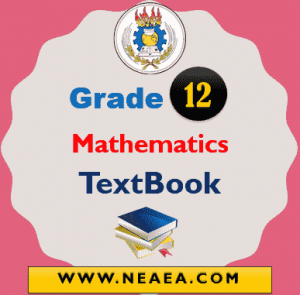# Ethiopian Grade 12 Mathematics Textbook For Students [PDF]

Ethiopian Grade 12 Mathematics Textbook For Students [PDF]. Download free PDF for grade 12 maths book provided by the Ethiopian Ministry Of Education (MOE).

The Grade 12 Mathematics Textbook consists of 9 units for For Natural Science Students and Social Science Students. The Maths subjects in Grade 12 comes under Common Courses therefore, students from another group also have this subject in common. After each unit, the book has Key terms, Summary, and Review Exercises.## Ethiopian Grade 12 Mathematics Student Textbook

Mathematics learning at the second cycle of secondary education (Grades 12) should contribute to the students’ growth into good, balanced, and educated individuals and numbers of society. At this cycle, they should acquire the necessary mathematical knowledge and develop skills and competencies needed in their further studies.

Content

Unit 1: Sequences and Series

• 1.1 Sequences
• 1.2 Arithmetic sequences and geometric sequences
• 1.3 The sigma notation and partial sums
• 1.4 Infinities series
• 1.5 Applications of arithmetic progressions and geometric progressions

Unit 2: Introduction to Limits and Continuity

• 2.1 Limits of sequences of numbers
• 2.2 Limits of functions
• 2.3 Continuity of a function
• 2.4 Exercises on applications of limits

Unit 3 Introduction to Differential Calculus

• 3.1 Introduction to Derivatives
• 3.2 Derivatives of some functions
• 3.3 Derivatives of combinations and compositions of functions

Unit 4 Applications of Differential Calculus

• 4.1 Extreme values of functions
• 4.2 Minimization and maximization problems
• 4.3 Rate of change

Unit 5 Introduction to Integral Calculus

• 5.1 Integration as reverse process of differentiation
• 5.2 Techniques of integration
• 5.3 Definite integrals, area and fundamental theorem of calculus
• 5.4 Applications of Integral calculus

Unit 6 Three Dimensional Geometry and Vectors in Space (For Natural Science Students)

• 6.1 Coordinate axes and coordinate planes in space
• 6.2 Coordinates of a point in space
• 6.3 Distance between two points in space
• 6.4 Mid-point of a line segment in space
• 6.5 Equation of sphere
• 6.6 Vectors in space

Unit 7 Mathematical Proofs (For Natural Science Students)

• 7.1 Revision on logic
• 7.2 Different types of proofs
• 7.3 Principle and application of mathematical induction

Unit 8 Further on Statistics (For Social Science Students)

• 8.1 Sampling techniques
• 8.2 Representation of data
• 8.3 Construction and interpretation of graphs
• 8.4 Measures of central tendency and measures of variability
• 8.5 Analysis of frequency distributions
• 8.6 Use of cumulative frequency curves

Unit 9 Mathematical Applications for Business and consumers (For Social Science Students)